Next: NCEPatm_2.m Up: Subsidiary calculation functions Previous: ET_objective.m   Contents

## lifton2006sp.m

Syntax:

scalingfactor = lifton2006sp(h,Rc,S);


Calculates the geographic scaling factor for cosmogenic-nuclide production for particular cutoff rigidity and altitude according to the scheme in:

Lifton N.A., Bieber J.W., Clem J.M., Duldig M.L., Evenson P., Humble J.E., Pyle R., 2005. Addressing solar modulation and long-term uncertainties in scaling secondary cosmic rays for in situ cosmogenic nuclide applications. Earth and Planetary Science Letters, v. 239, pp. 140-161.

The input arguments are h, atmospheric pressure (hPa), Rc, cutoff rigidity (GV), and S, the solar modulation factor (nondimensional; see the source paper for details). Accepts vector arguments.

This function:

Converts atmospheric pressure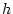(hPa) to atmospheric depth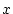(g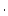cm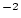) by multiplying by 1.0197.

Assigns cutoff rigidities below 1.907 GV a value of 1.907.

Calculates the scaling factor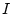(Lifton's nomenclature) using Equation (4) in the source paper: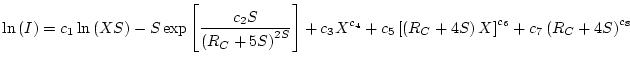(40)

where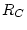is the cutoff rigidity,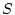is the solar modulation factor, and the constants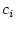are: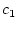1.8399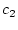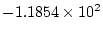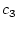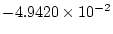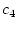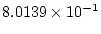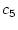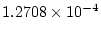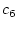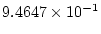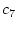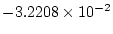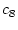1.2688

Accepts either scalars or vectors of equal sizes for all the input arguments. Returns either a scalar or a vector of the appropriate size.Next: NCEPatm_2.m Up: Subsidiary calculation functions Previous: ET_objective.m   Contents
2007-11-13